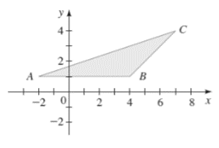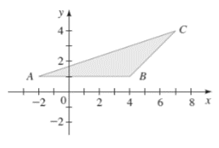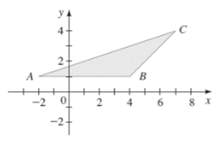# The area of the triangle shown in the figure,### Precalculus: Mathematics for Calcu...

6th Edition
Stewart + 5 others
Publisher: Cengage Learning
ISBN: 9780840068071### Precalculus: Mathematics for Calcu...

6th Edition
Stewart + 5 others
Publisher: Cengage Learning
ISBN: 9780840068071

#### Solutions

Chapter 1.8, Problem 38E
To determine

## To calculate: The area of the triangle shown in the figure,Expert Solution

The area of the triangle is 9 sq. units .

### Explanation of Solution

Given information:

The figure,Formula used:

Area of a triangle is half into the product of its base and height. So, if b is the base and h is its height, area of triangle is calculated as,

A=12bh

Calculation:

Consider the given figure,In the above figure, it is observed that the base of triangle is measured as 6 units and its height is 3 units.

Recall that the area of a triangle is half into the product of its base and height. So, if b is the base and h is its height, area of triangle is calculated as,

A=12bh

Apply it,

A=12bh=1263=1218=9 sq. units

Thus, area of the triangle is 9 sq. units .

### Have a homework question?

Subscribe to bartleby learn! Ask subject matter experts 30 homework questions each month. Plus, you’ll have access to millions of step-by-step textbook answers!